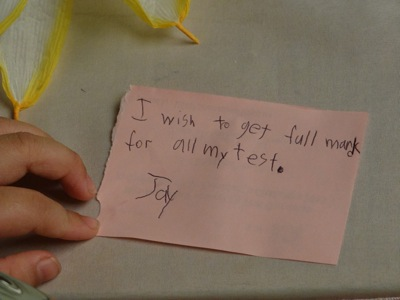## Tuesday, September 17, 2013

### Studying for Math Test

Date:  Sep 17, 2013

Hey, I have to be careful using the word "Test" or "Exam" for Primary One students.  Ha Ha Ha Ha.

P1 & P2 not suppose to have "Test" or "Exam".  They have what they so called "Holistic Assessment".

Aiya, MOE is really… really…. "Holistic Assessment" has to be tested under a "Test" or "Exam" format.  So, it is still a "Test" or "Exam" la.

After we been thru Term 2 and Term 3, although they did not say when exactly, we know the "Test" is going to be on the first or second week.

There is nothing much I can do about Chinese and English.  So, my job is to make sure Jay Jay understand the concept of Multiplications and Divisions.

The other day, I shared how I train Mr. Jay to do Multiplication by self creating a Multiplication Table.

Also, I blogged about how a Geeky dad trained his son on Divisions Concept & Prime Numbers.

Let's put all these concepts into test.  My test.

HaHaHa

It is just the same as what every parents would do, buy some extra assessment book for him to do.  Then correct it and teach him the taught process.

So, let's start from the book from the left and one by one he finished the Multiplication and Divisions chapter in a few days.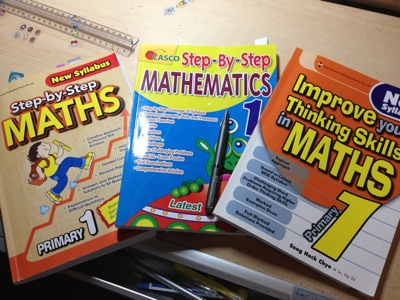Initially on the first book.  It is a assessment on how they think, and what they know from the class.

Seldom you get 100% right.  Given that this is the first time, parent steps in to teach him.

Like Question #9, I have to teach him that, always use the correct operations based on information given.  If there are two piece of info given, he has to put the information in the left side of the equation, Instead of putting it anywhere.

For Example, here you know you got total of 25 balloons, and he bought three packets of 8 balloons which total to 24.  So, He must know and learned how to put 25 and 24 on the left side and make use of "Subtraction" to find answer.

(Ignore Question #10, I think the question itself is in question).  My son answer correctly, because she is short of 3 pencil, and how many does see need, which is 3.  What kind of questions is this?

So, sometimes assessment book also got lousy questions or wrong question.  Must be careful and explain to them.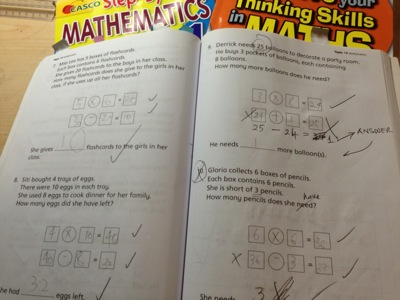He really hates this kind of question.  Because it is like writing a English journal, he said.  He sure kalang kaput when answer this kind of question, and like daddy, his English need a lot of polishing.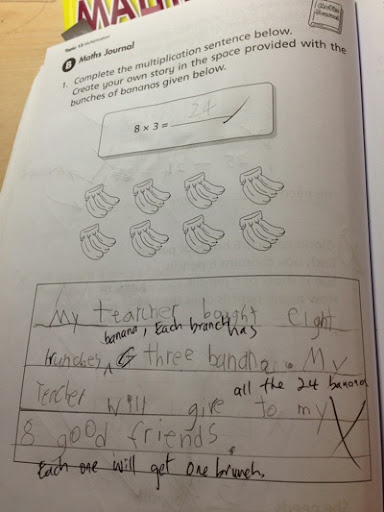Sometimes you get a few of challenging questions.  But it is fun, and that is how he learned, by having fun!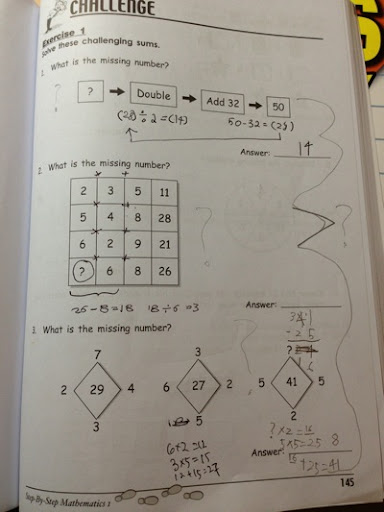OK.  Now, we come to the main topic of this blog entry.

1.  When you see questions like this where it has space between questions, that means he has to do the working and find the answers.

2.  Although he is P1, but you know that MOE promotes Model drawings.  So, you can start teaching him Model.

3.  Let him visualise the problem by drawings, and then, at the side produce the working.

4.  If you do not know what is Model, I suggest you can go learn it in some talks, or tutorial session.  I actually paid to attend a parent tuition on Model drawings.  I learned a great deal.  Those questions previously I thought need to use Algebra to solve, can all be solved by simple Model drawings and visualising the Model.

5.  In primary schools, they are going to use a lot of Model drawings to solve their problems.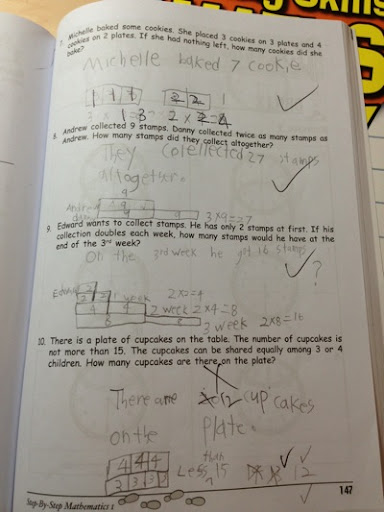For example.  This questions here.

Andrew collected 9 stamps.

Danny collected "TWICE" as many stamps as Andrew.

How many stamps did they collected all together.

So, for Andrew, you draw a rectangular of 9.  Then, on the below of the first rectangular, you draw two equal size rectangular, as Danny collected twice.  Fill in the "9".

So, you know you need to solve 3 x 9 = 27.

The other questions is ...

Edward has 2 stamps at first.

So each week, he doubles his collection.

So, 1st week, you draw two rectangular of the same size.

2nd week, you draw the same rectangular of the same size than the week before.

In this case, you add up the 2+2=4, and fill in 4 in both rectangular.

And do the same for last week.  You fill in 8's in both rectangular.

So, you know to find total stamps he has for 3rd week, he has to do 2 x 8 = 16.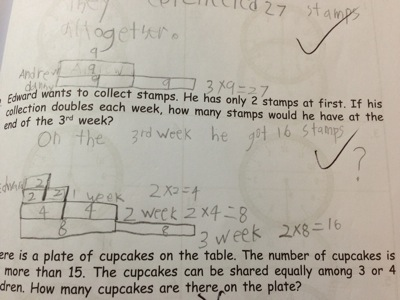I love doing his math together with him.

I never learned Model drawing before.  Before that, I solve everything using Algebra.

But now, since I have some prior knowledge of drawing the Models, I am using it to solve the question.

And I love it because it is also a challenge for me to see how to draw the CORRECT MODEL and the child can take a look and find out what is the answer quickly.

HaHaHaHaHa.

So, for teachers/adults, I drew 5 squares.  And I know each square is \$3 each.

Immediately, under the teacher squares, I drew a rectangular which equal to 5x square.  That will be my 5 students.  So, I drew 3 big rectangular, which equal to 15 students.

Each rectangular, I marked 5x\$2 to represent a group of 5 students.

So, for each group of 5 students, I SHADED one square in the teacher/adult square.  Because, every 5 children get a free adult ticket.

So, you got 3 groups of 5, then, you SHADED 3 squares.

Fill in the dollar value \$3.

And now, you can solve the total admission fee needed which is total to \$36.

Jay did this wrong.  But after he saw the Model drawing, he immediately understood.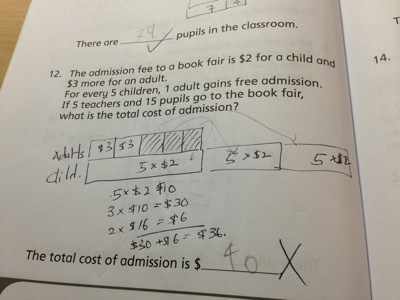Jay Jay is very lazy.  When he did the last book on Sunday.  He did not do any workings at all and straight away write the answers.

For both chapter in this suppose to be hardest book, he score all correct.

Well, after the training from his school homework, and the previous two books, he becomes better.

And he felt very proud of completing the last book with flying colours.  HaHaHaHaHa.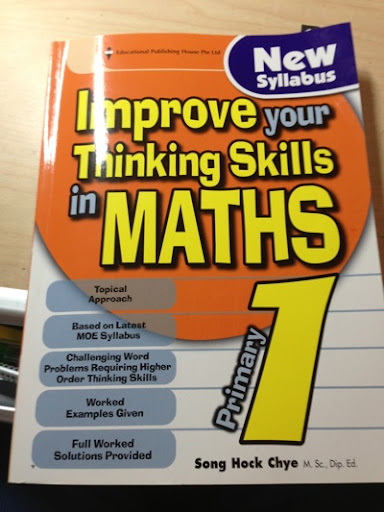Another one.  I love this sort of question as this is the typical solving Math using Model drawing question.

So, for this question.

Because Box A has 4 more than box B.

1.  So, you draw the rectangular of A which is longer than B.

2.  Both rectangular has to draw side by side vertically.

3.  Then, you EQUATE THE PARTS.  Mean, you draw a line so that the rectangular A has equal parts same as rectangular B.

4.  Fill in "4" in the smaller rectangular there.

5.  Next clues is Box B has 6 FEWER than Box C.  So, you know Box C going to be longer than Box A and B.

6.  So, you draw a longest box there.  Then, you EQUATE THE PARTS AGAIN.

7.  You can fill in 6 or further equate the parts as Box A, so that you can fill in 4 and 2 in separate equate parts.

8.  How many marbles in Box C?

9.  So, you take 25 subtract those extra one, which is 25 - 4 - 6 = 15

10.  Then, you know the for P1, their division is merely draw all the 15 objects there and CIRCLE equally.

11.  So, the answer of the missing square for A, B and C (which is all equal) is "5".

12.  So, Box C has 5 + 6 = 11.

I love the Model drawings, as it really helps to constructs your math concept and your thought process in a very organised manner.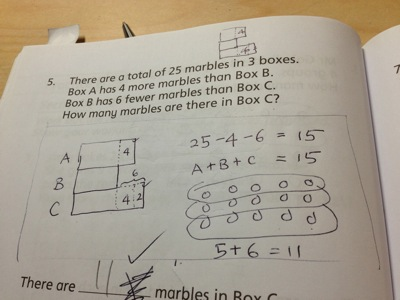All the divisions you can simply draw the total objects and figure out how to circle the groups.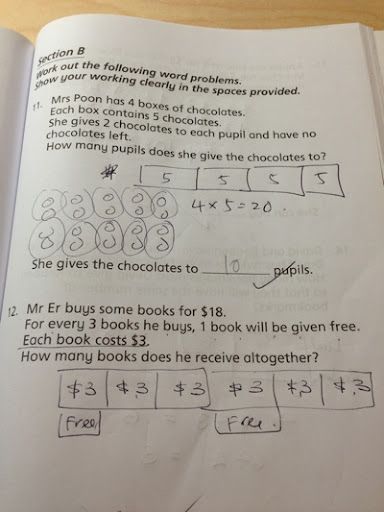This is the last questions.  And it seems that he has read the questions very carefully and that is why he can score 100 in both Multiplications and Divisions in this book.

Good job Jay.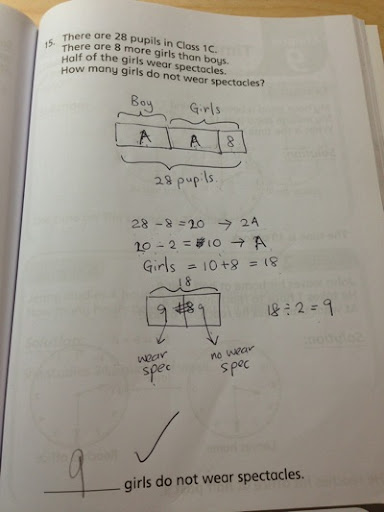No wonder when we go Garden by the Bay yesterday, he wrote this on his wishing card.He is such a confident kid.  Now, only his carelessness can stop him from getting full marks.  hahahahaha

Good luck my son.  You can do it.  Even you don't get full marks, I know you has learned well in both the multiplication and division concepts.  Your foundation has been fixed.